# Predict if the solutions of the following salts are neutral, acidic or basic:

Question:

Predict if the solutions of the following salts are neutral, acidic or basic:

$\mathrm{NaCl}, \mathrm{KBr}, \mathrm{NaCN}, \mathrm{NH}_{4} \mathrm{NO}_{3}, \mathrm{NaNO}_{2}$ and $\mathrm{KF}$

Solution:

(i) NaCl: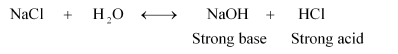Therefore, it is a neutral solution.

(ii) KBr: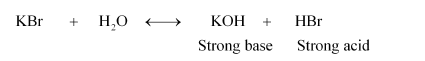Therefore, it is a neutral solution.

(iii) NaCN: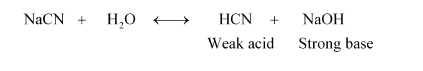Therefore, it is a basic solution.

(iv) $\mathrm{NH}_{4} \mathrm{NO}_{3}$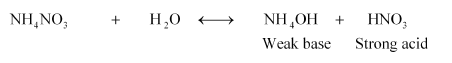Therefore, it is an acidic solution.

(v) NaNO2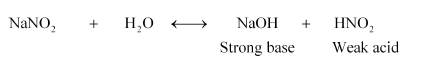Therefore, it is a basic solution.

(vi) KF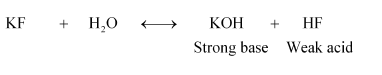Therefore, it is a basic solution.